$\newcommand{\NN}{\mathbb{N}} \newcommand{\CC}{\mathbb{C}} \newcommand{\GG}{\mathbb{G}} \newcommand{\LL}{\mathbb{L}} \newcommand{\PP}{\mathbb{P}} \newcommand{\QQ}{\mathbb{Q}} \newcommand{\RR}{\mathbb{R}} \newcommand{\VV}{\mathbb{V}} \newcommand{\ZZ}{\mathbb{Z}} \newcommand{\FF}{\mathbb{F}} \newcommand{\KK}{\mathbb{K}} \newcommand{\UU}{\mathbb{U}} \newcommand{\EE}{\mathbb{E}} \newcommand{\Aa}{\mathcal{A}} \newcommand{\Bb}{\mathcal{B}} \newcommand{\Cc}{\mathcal{C}} \newcommand{\Dd}{\mathcal{D}} \newcommand{\Ee}{\mathcal{E}} \newcommand{\Ff}{\mathcal{F}} \newcommand{\Gg}{\mathcal{G}} \newcommand{\Hh}{\mathcal{H}} \newcommand{\Ii}{\mathcal{I}} \newcommand{\Jj}{\mathcal{J}} \newcommand{\Kk}{\mathcal{K}} \newcommand{\Ll}{\mathcal{L}} \newcommand{\Mm}{\mathcal{M}} \newcommand{\Nn}{\mathcal{N}} \newcommand{\Oo}{\mathcal{O}} \newcommand{\Pp}{\mathcal{P}} \newcommand{\Qq}{\mathcal{Q}} \newcommand{\Rr}{\mathcal{R}} \newcommand{\Ss}{\mathcal{S}} \newcommand{\Tt}{\mathcal{T}} \newcommand{\Uu}{\mathcal{U}} \newcommand{\Vv}{\mathcal{V}} \newcommand{\Ww}{\mathcal{W}} \newcommand{\Xx}{\mathcal{X}} \newcommand{\Yy}{\mathcal{Y}} \newcommand{\Zz}{\mathcal{Z}} \newcommand{\al}{\alpha} \newcommand{\la}{\lambda} \newcommand{\ga}{\gamma} \newcommand{\Ga}{\Gamma} \newcommand{\La}{\Lambda} \newcommand{\Si}{\Sigma} \newcommand{\si}{\sigma} \newcommand{\be}{\beta} \newcommand{\de}{\delta} \newcommand{\De}{\Delta} \renewcommand{\phi}{\varphi} \renewcommand{\th}{\theta} \newcommand{\om}{\omega} \newcommand{\Om}{\Omega} \renewcommand{\epsilon}{\varepsilon} \newcommand{\Calpha}{\mathrm{C}^\al} \newcommand{\Cbeta}{\mathrm{C}^\be} \newcommand{\Cal}{\text{C}^\al} \newcommand{\Cdeux}{\text{C}^{2}} \newcommand{\Cun}{\text{C}^{1}} \newcommand{\Calt}{\text{C}^{#1}} \newcommand{\lun}{\ell^1} \newcommand{\ldeux}{\ell^2} \newcommand{\linf}{\ell^\infty} \newcommand{\ldeuxj}{{\ldeux_j}} \newcommand{\Lun}{\text{\upshape L}^1} \newcommand{\Ldeux}{\text{\upshape L}^2} \newcommand{\Lp}{\text{\upshape L}^p} \newcommand{\Lq}{\text{\upshape L}^q} \newcommand{\Linf}{\text{\upshape L}^\infty} \newcommand{\lzero}{\ell^0} \newcommand{\lp}{\ell^p} \renewcommand{\d}{\ins{d}} \newcommand{\Grad}{\text{Grad}} \newcommand{\grad}{\text{grad}} \renewcommand{\div}{\text{div}} \newcommand{\diag}{\text{diag}} \newcommand{\pd}{ \frac{ \partial #1}{\partial #2} } \newcommand{\pdd}{ \frac{ \partial^2 #1}{\partial #2^2} } \newcommand{\dotp}{\langle #1,\,#2\rangle} \newcommand{\norm}{|\!| #1 |\!|} \newcommand{\normi}{\norm{#1}_{\infty}} \newcommand{\normu}{\norm{#1}_{1}} \newcommand{\normz}{\norm{#1}_{0}} \newcommand{\abs}{\vert #1 \vert} \newcommand{\argmin}{\text{argmin}} \newcommand{\argmax}{\text{argmax}} \newcommand{\uargmin}{\underset{#1}{\argmin}\;} \newcommand{\uargmax}{\underset{#1}{\argmax}\;} \newcommand{\umin}{\underset{#1}{\min}\;} \newcommand{\umax}{\underset{#1}{\max}\;} \newcommand{\pa}{\left( #1 \right)} \newcommand{\choice}{ \left\{ \begin{array}{l} #1 \end{array} \right. } \newcommand{\enscond}{ \left\{ #1 \;:\; #2 \right\} } \newcommand{\qandq}{ \quad \text{and} \quad } \newcommand{\qqandqq}{ \qquad \text{and} \qquad } \newcommand{\qifq}{ \quad \text{if} \quad } \newcommand{\qqifqq}{ \qquad \text{if} \qquad } \newcommand{\qwhereq}{ \quad \text{where} \quad } \newcommand{\qqwhereqq}{ \qquad \text{where} \qquad } \newcommand{\qwithq}{ \quad \text{with} \quad } \newcommand{\qqwithqq}{ \qquad \text{with} \qquad } \newcommand{\qforq}{ \quad \text{for} \quad } \newcommand{\qqforqq}{ \qquad \text{for} \qquad } \newcommand{\qqsinceqq}{ \qquad \text{since} \qquad } \newcommand{\qsinceq}{ \quad \text{since} \quad } \newcommand{\qarrq}{\quad\Longrightarrow\quad} \newcommand{\qqarrqq}{\quad\Longrightarrow\quad} \newcommand{\qiffq}{\quad\Longleftrightarrow\quad} \newcommand{\qqiffqq}{\qquad\Longleftrightarrow\qquad} \newcommand{\qsubjq}{ \quad \text{subject to} \quad } \newcommand{\qqsubjqq}{ \qquad \text{subject to} \qquad }$

Inpainting using Variational Regularization

# Inpainting using Variational Regularization

This numerical tour explores the use of variational energies (Sobolev, total variation) to regularize the image inpaiting problem.

## Contents

Here we consider inpainting of damaged observation without noise.

## Installing toolboxes and setting up the path.

You need to download the following files: signal toolbox and general toolbox.

You need to unzip these toolboxes in your working directory, so that you have toolbox_signal and toolbox_general in your directory.

For Scilab user: you must replace the Matlab comment '%' by its Scilab counterpart '//'.

Recommandation: You should create a text file named for instance numericaltour.sce (in Scilab) or numericaltour.m (in Matlab) to write all the Scilab/Matlab command you want to execute. Then, simply run exec('numericaltour.sce'); (in Scilab) or numericaltour; (in Matlab) to run the commands.

Execute this line only if you are using Matlab.

getd = @(p)path(p,path); % scilab users must *not* execute this


Then you can add the toolboxes to the path.

getd('toolbox_signal/');
getd('toolbox_general/');


## Missing Pixels and Inpainting

Inpainting corresponds to filling holes in images.

First we load the image $$f_0 \in \RR^N$$ of $$N=n\times n$$ to be inpainted.

name = 'cameraman';
n = 256;
f0 = rescale( load_image(name, n) );


Display the original image.

clf;
imageplot(f0);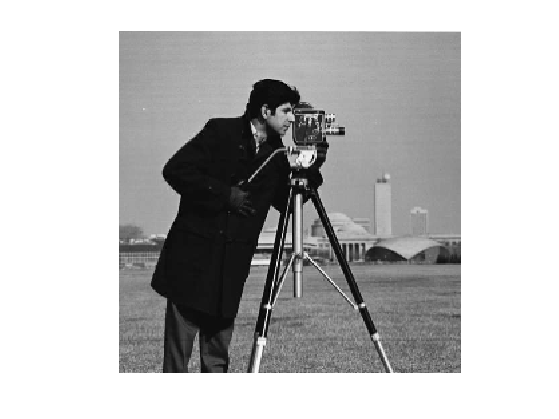Ratio of removed pixels.

rho = .7;


Construct a random mask $$\Ga = \chi_{\Om}$$ so that $$\Ga_i=0$$ for removed pixels $$i \notin \Om$$, and $$\Ga_i=1$$ for kept pixels $$i \in \Om$$.

Gamma = rand(n)>rho;


We create the masking operator $$\Phi$$ which is a diagonal operator: $(\Phi f)_i = \Ga_i f_i$

Phi = @(f)f.*Gamma;


Compute the damaged observation $$y=\Phi(f_0)$$ (no noise is added).

y = Phi(f0);


Display the observations.

clf;
imageplot(y);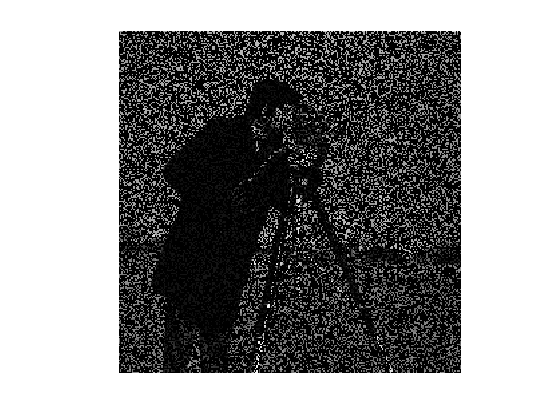## Sobolev Impainting

We solve the inpainting problem by minimzing the Sobolev norm of the image under the constraint of matching the observation $f^\star = \uargmin{ \Phi(f) = y } E(f) = \norm{\nabla f}^2$ where $$\nabla$$ is a finite difference approximation of the gradient.

It can be shown that the solution to this problem is an harmonic function with prescribed boundary condition $\forall i \notin \Om, \quad (\Delta f^\star)_i=0 \qandq \forall i \in \Om, \quad f^\star_i = y_i.$

This problem requires the constrained minimization of a smooth function, it can thus be solved using a projected gradient descent $f^{(\ell+1)} = \Pi \pa{ f^{(\ell)} + \tau \Delta(f^{(\ell)}) }$ where $$\Pi$$ is the orthogonal projector on the constraint $$y=\Phi f$$ $(\Pi f)_i = \choice{ y_i \qifq i \in \Om, \\ f_i \qifq i \notin \Om, \\ }$

Pi = @(f)f.*(1-Gamma) + y.*Gamma;


Here $$\Delta = -\nabla^* \circ \nabla = \text{div} \circ \nabla$$ is the gradient of the Sobolev energy $$E$$.

Delta = @(f)div(grad(f));


For convergence, the gradient descent step size should satisfy: $\tau<\frac{2}{\norm{\Delta}}=\frac{1}{4}$

tau = .8/4;


Exercice 1: (check the solution) Perform the projected gradient descent. Record in a variable E the evolution of the Sobolev energy $$E$$.

exo1;Display the decay of the energy $$E(f^{(\ell)})$$ with the iterations.

clf;
plot(E); axis('tight');
set_label('Iteration #', 'E');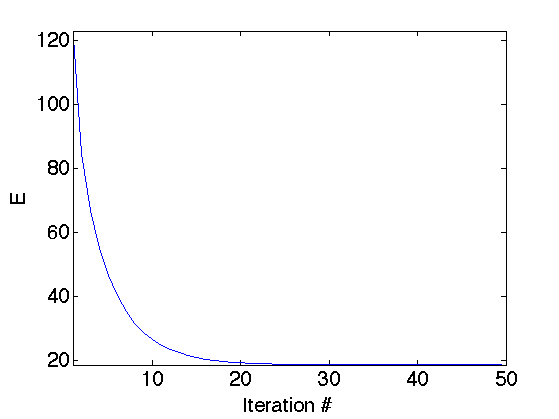Display the result.

clf;
imageplot(f, strcat(['Inpainted, SNR=' num2str(snr(f0,f),3) 'dB']));## Inpainting with TV Regularization

A non-linear prior replaces the Sobolev energy by the TV norm, that tends to better reconstruct edges. Here we use a smoothed TV norm to avoid convergence issue with gradient descent algorithms.

The smoothed TV norm reads: $J_\epsilon(f) = \sum_x \sqrt{\norm{ \nabla f(x) }^2+\epsilon^2}$

We use a projected gradient descent to solve this problem $f^{(\ell+1)} = \Pi \pa{ f^{(\ell)} + \tau G_\epsilon(f^{(\ell)}) }$ where $$G_\epsilon$$ is the gradient of $$J_\epsilon$$, that is defined as $G_\epsilon(f) = -\text{div} N_\epsilon( \nabla f )$ where $$N_\epsilon$$ is the following normalization operator $N_\epsilon(u)_i = \frac{u_i}{ \sqrt{\norm{u_i}^2 + \epsilon^2} }$ that is applied to any vector field $$u=(u_i)_i \in \RR^{N \times 2}$$ for $$u_i \in \RR^2$$.

Regularization parameter $$\epsilon$$ for the TV norm

epsilon = 1e-2;


Define the normalization operator.

Amplitude = @(u)sqrt(sum(u.^2,3)+epsilon^2);
Neps = @(u)u./repmat(Amplitude(u), [1 1 2]);


The step size $$\tau$$, should satisfy $\tau<\frac{\epsilon}{4}.$

tau = .9*epsilon/4;


Define the gradient of $$J$$

G = @(f)-div(Neps(grad(f)));


Exercice 2: (check the solution) Perform the projected gradient descent. Record in a variable J the evolution of the TV energy $$J_\epsilon$$.

exo2;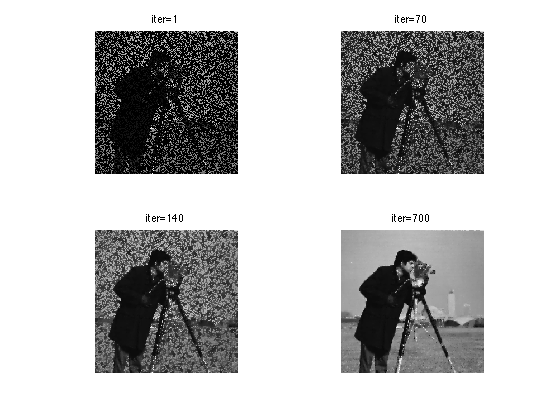Display the result.

clf;
imageplot(clamp(f), strcat(['SNR=' num2str(snr(f0,f),3) 'dB']));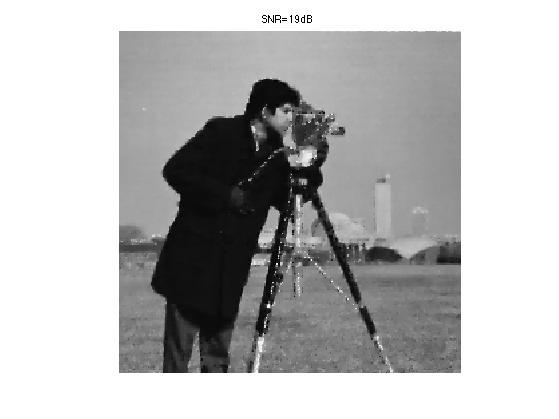Display the evolution of the TV norm $$J_\epsilon$$.

clf;
plot(J);
axis('tight');
set_label('Iteration #', 'J_\epsilon');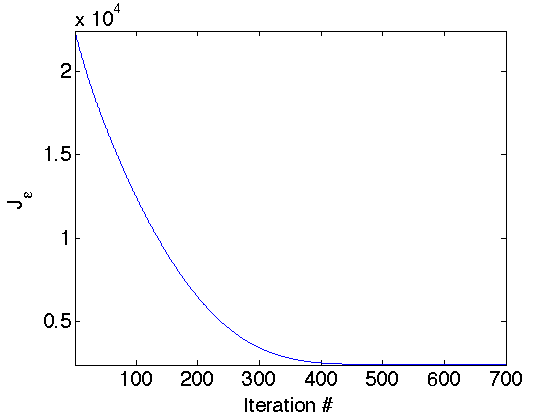## Inpainting with non-random mask

Inpainting can be used to remove objects in pictures.

n = 256;
f0 = load_image('parrot', n);
f0 = rescale( sum(f0,3) );


Display it.

clf;
imageplot(f0);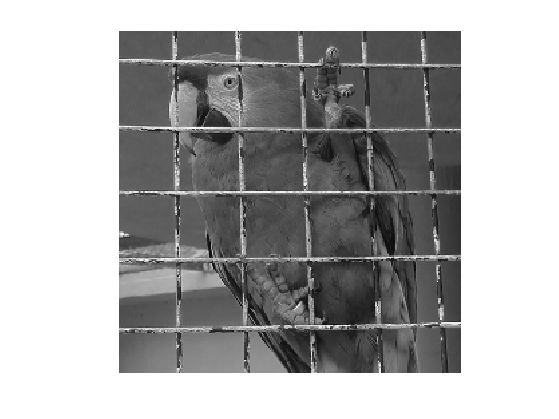Gamma = load_image('parrot-mask', n);
Gamma = double(rescale(Gamma)>.5);


Masking operator $$\Phi$$.

Phi = @(f)f.*Gamma;


Observation $$y=\Phi(f_0)$$.

y = Phi(f0);


Display it.

clf;
imageplot(y);Exercice 3: (check the solution) Perform Sobolev inpainting on this image.

exo3;Exercice 4: (check the solution) Try other methods to solve this inpainting problem. You can for instance have a look on the numerical on sparsity for deconvolution and inpainting.

exo4;